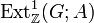# Second cohomology group for trivial group action between additive groups of ring of integers localized at sets of primes

The goal of this page is to discuss the second cohomology group for trivial group action$H^2(G;A)$ where both$G$ and$A$ are additive groups of rings of integers localized at sets of primes. Explicitly, there are subsets$\pi_1,\pi_2$ of the set of all prime numbers such that$G = \mathbb{Z}[\pi_1^{-1}]$ and$A = \mathbb{Z}[\pi_2^{-1}]$.

## Computation of the group

We use the formula for second cohomology group for trivial group action of abelian group in terms of Schur multiplier and abelianization (which is a special case of the formula for second cohomology group for trivial group action in terms of Schur multiplier and abelianization), namely the following short exact sequence:$0 \to \operatorname{Ext}^1_{\mathbb{Z}}(G,A) \to H^2(G;A) \stackrel{\operatorname{Skew}}{\to} \operatorname{Hom}(\bigwedge^2G,A) \to 0$

where$\bigwedge^2G = M(G)$ is the exterior square of$G$ and also coincides with the Schur multiplier of$G$.

Note that since locally cyclic implies epabelian, the Schur multiplier of$G$ is the trivial group, and all extensions are themselves abelian groups. In particular,$\bigwedge^2G = 0$, and we get:$H^2(G;A) \cong \operatorname{Ext}^1_{\mathbb{Z}}(G,A)$

Thus, the computation of$H^2(G;A)$ is equivalent to the computation of$\operatorname{Ext}^1_{\mathbb{Z}}(G;A)$, and the elements of these classify the congruence classes of group extensions, all of which are abelian.

We now turn to an explicit description in terms of the sets$\pi_1$ and$\pi_2$. First, define$\pi_3$ as the set of all primes in$\pi_1$ that are not in$\pi_2$. Now:

• Let$R$ be the external direct product of the additive groups of p-adic integers for all$p \in \pi_3$. Note that if$\pi_3$ is empty, we take$R$ as the trivial group.
• Recall that there is a natural embedding of$\mathbb{Z}[\pi_2^{-1}]$ inside each of the direct factors of$R$ (we begin with the natural embedding of$\mathbb{Z}$ inside$\mathbb{Z}_{(p)}$, the p-adics, then extend to an embedding of all of$\mathbb{Z}[\pi_2^{-1}]$ using the fact that$\mathbb{Z}_{(p)}$ is$\pi_2$-powered. Let$S$ be the image of the diagonal embedding in$R$ arising from each of these embeddings.
• The group$H^2(G;A) \cong \operatorname{Ext}^1_{\mathbb{Z}}(G,A)$ that we are trying to compute is$R/S$.

Some simple cases are described below:

Case Description of$H^2(G;A) \cong \operatorname{Ext}^1_{\mathbb{Z}}(G,A)$$\pi_1 \subseteq \pi_2$, in other words, the base of the extension has all the powering that the extension group does, and possibly more. trivial group$\pi_1 = \{ p \}, \pi_2 = \{ \}$ quotient of additive group of p-adic integers by group of integers, i.e., the quotient$\mathbb{Z}_{(p)}/\mathbb{Z}$$\pi_1$ is the set of all primes,$\pi_2 = \{ \}$ quotient of profinite completion of the integers by the integers, i.e., the group$\hat{\mathbb{Z}}/\mathbb{Z}$.

## Elements

Basically, we use the element of the$p$-adic group to describe what$p^{th}$ root to adjoin at each stage.